﻿ A Sobolev Space Inroad to Riemann IntegrabilityPublications are Open
Access in this journal
Article Versions
Export Article
• Normal Style
• MLA Style
• APA Style
• Chicago Style
Research Article
Open Access Peer-reviewed

### A Sobolev Space Inroad to Riemann Integrability

Nassar H. S. Haidar
Turkish Journal of Analysis and Number Theory. 2020, 8(2), 34-38. DOI: 10.12691/tjant-8-2-3
Received June 04, 2020; Revised July 05, 2020; Accepted July 13, 2020

### Abstract

A conditioned equivalence is proved for a certain weighted Sobolev space to the space of Riemann integrable functions. An equivalence representing a new result that not only asserts the sufficiency (but non-necessity) nature of bounded variation of functions for their Riemann integrability, but also reveals a potential for some novel computational findings.

### 1. Introduction

The transparent classical definition of the Riemann sum type of integral, see e.g. 1, was shown by Graves in 2, to admit an easy extension to a following integration of Banach space-valued functions, 3. Indeed, let f: I → X be given, where X is a Banach space with the norm ‖ .X and the compact interval I = [a,b] R = (-∞,∞) is endowed with a length measure λ. f(x) is said to be Riemann integrable and γ X its Riemann integral if for every ε > 0, a constant gauge δ R =[0,∞), such that for every δ-fine K-partition {xi , Ii : i Γ}, see e.g. 3, the inequality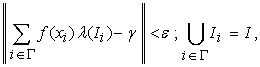holds.

Moreover, if in the previous statement δ R is replaced by δ:I →R, f would be Henstock-Kurzweil, 4, integrable, whereas if both δR and K- partition in the previous statement are respectively replaced by δ : I → R, and M- partition, 3, f becomes MacShane, 5, integrable. The same approach was followed by Bochner, in 6, for Lebesgue integrability in Banach spaces, i.e. when the above λ is a Lebesgue measure, to introduce the Bochner integral.

Lebesgue integrability happens also, however, to be a defining operation for all Sobolev spaces, see e.g. 7, and Riemann integrability in Sobolev spaces may sound rather unusual. The goal of this note, is to demonstrate that a linearly weighted Sobolev space can serve, nonetheless, as a natural space for Riemann integrable functions. The obtained result not only constitutes an addition to the existing literature, e.g. 3, on vector-valued integration, but also reveals a potential for some novel computational findings.

### 2. Main Result

The space of ρ-weighted Riemann integrable functions over a closed interval I,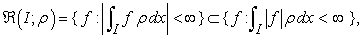(1)

where both integrals are conceived in the Riemann sense, is well known to be seminormed, see e.g. 1, 7. A seminorm on a functional vector space X, with f,g X and c ℂ, is a function ξ: X→R with the properties:

i) ξ(cf) = |c| ξ(f), absolute homogeneity,

ii) ξ(f+g) ≤ ξ(f) + ξ(g), subadditivity.

It is clear that Ker(ξ) = {f X: ξ(f)=0} is a subspace of X. Moreover, a seminorm ξ becomes a norm υ if Ker(ξ)={0}. Hence a norm υ: X → R is a seminorm with the additional property:

iii) υ(f) ≥ 0, fX, with the equality if f ≡ 0, point separability.

Both integrals in (1), namely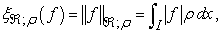(2)

or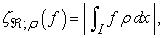(3)

can be seminorms for (I;ρ), because they happen to satisfy the previous properties (i) and (ii) but may violate (iii). Indeed ξ; (f) = 0 if f = 0 only a.e., that is when f ≈ 0, while ζ; (f) = 0, when e.g.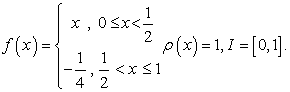This makes ℜ(I;ρ) a vector space of equivalence classes of ρ-weighted Riemann integrable functions on I, where f can be equivalent to g iff f = g a.e. Nonetheless, by application of the Riesz-Fischer theorem, 1, 7, it can readily be proved that (I;ρ) is complete under the seminorm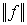; , i.e. (I;ρ) is a Banach space.

Since (I;ρ) L¹(I;ρ), one should not confuse ξ; (f) =; with the seminorm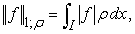(4)

of the p =1 case of Lp spaces, in which the integral is conceived in the Lebesgue sense. Note moreover that L¹ happens to be unusual among the Lp spaces in that it is not reflexive. Incidentally, conventional ρ-weighted Sobolev spaces Wp,k(I;ρ) are related to these Lp spaces and have additional structure viz.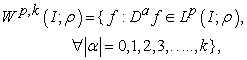(5)

as they are endowed with the norm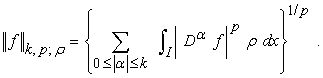(6)

Here, the D f derivatives, up to a given k-order, are understood in a weak sense, to make the Wp,k(I;ρ) space complete, thus a Banach space.

Definition 1. (Functions of bounded variation, 8, 9). Let us subdivide the I=[a,b] interval arbitrarily into n subintervals to form a finite partition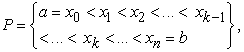(7)

with the sum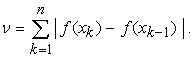(8)

A function f: I→R is of bounded variation (BV), or f BV(I), is characterized by the norm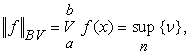(9)

and is defined when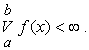(10)

Definition 2. (Hölder continuous functions). f : I →R is said to be Hölder continuous (HC) if α > 0 such that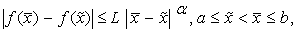(11)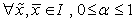, and L is some > 0 constant.

The special case of α = 1 corresponds to the case of Lipschitz continuous (LC) functions; and it is well known that Lipschitzianity is weaker than continuous differentiability (CD), and stronger than uniform continuity (UC) or continuity (C).

As for the (I;ρ) space of interest to this work, its related L¹(I;ρ) space naturally invokes W1,1(I;ρ), which is known, 5, 7, to be isomorphic to the space of functions of BV, endowed with the normBV , defined by (9-10). Hence W1,1(I;ρ) is a space of absolutely continuous functions on I or equivalence classes of ρ-weighted Lebesgue integrable functions that are a.e. absolutely continuous. It is a Banach space w.r.t. the norm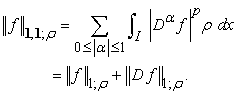(12)

Replacement of the Lebesgue integrability condition in the definition of W1,1(I;ρ) by Riemann integrability transforms it to an unconventional Riemann-Sobolev space W,1 (I;ρ) endowed with the norm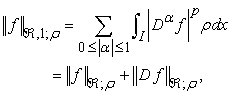(13)

where the index refers to integration in the Riemann sense of functions essentially in BV(I), isomorphic with W1,1(I;ρ).

To demonstrate then that,1; is a norm, we examine it against the previous three related properties. Although the second term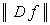; in (13) is always a seminorm since it annihilates constants, i.e. violates the norm property (iii), the first term; does not. This guarantees, incidentally, satisfaction of (iii) by the entire,1;. Property (i) is clearly satisfied by both components of,1; , then by,1; itself. It is also straight forward to prove that both; and; satisfy property (ii). To illustrate this, let I=[0,1], ρ(x)=1 on I and let f, g ∈ W,1(I;ρ) be f(x) = x and g(x)= -x. Clearly,;1 =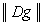;1 = 1 , while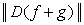;1 = 0, whence;1 +;1 >;1 is a satisfaction of the triangle inequality by;. The inequality,1 +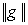,1 >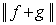,1 can similarly be established to confirm satisfaction of property (ii) by the sum,,1; , of these two terms.

Theorem 1. Let I = [a,b] ⊂ R, then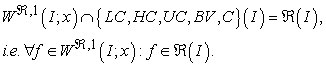(14)

Proof. Consider integration by parts of the Stieltjes integral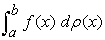, see e.g. 8, 10, via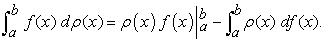(15)

On one hand, existence of either one of the Stielties integrals implies existence of the other, 11, with a satisfaction of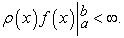(16)

On the other hand, classical analysis results, 4, 12, show that the left Stieltjes integral of (15) always exists, with both (15) and (16) valid, if

i) f(x) C(I) and ρ(x) BV(I),

ii) f(x) ℜ(I) and ρ(x) LC(I),

iii) f(x) α-HC(I) and ρ(x) β-HC(I); α + β >1, and the three statements are reflexive by replacement of f with ρ. Results that cohere with the following classical inclusion over I, 13,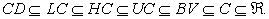(17)

Next assume in (15) that ρ(x) = x to reduce it to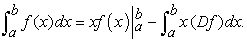(18)

Relation (18) happens remarkably to operate as a "functional quadrupole" with two inputs , ρ and f, and two outputs, (I) and W,¹(I;x). In this quadrupole, the possible {CD, LC, HC, UC, BV, C, ℜ}(I) spaces for ρ and f combine, according to the results (i)-(iii) and their reflections, in order to maintain the outputs fixed. In particular, (18) guarantees existence of f W,¹(I;x) simultaneously in any space of the set { LC, HC, UC, BV, C}(I), and not only in the isomorphic BV(I), when ρ(x) = x CD(I) = {CD ∩ LC ∩ HC ∩ UC ∩ BV ∩ C∩ℜ}(I).

Clearly therefore if f W,¹(I;x), then f ℜ(I), which is the required result.

We should underline here that BV of f(x) over I is not necessary, though sufficient for its Riemann integrability. The same is true if the BV condition is replaced by the stronger LC condition, 3 or 9. Moreover, LC(I) implies CD(I) a.e., and many functions can LC(I) while CD(I). So LC is weaker than CD, while being stronger than BV or C. Now it is time to state the important definition that follows, on Riemann integrability in a linearly-weighted Riemann-Sobolev space.

Definition 3. f : IW,¹(I;x) is Riemann integrable in W,¹(I;x), whenever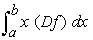exists.

Remark 1. It should be noted that satisfaction of x f(x)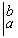< ∞ alone in (18) does not necessarily mean existence of the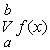, i.e. that f(x) is of BV. But with added integrability of Df(x) over I, w.r.t. the x weight, f(x) becomes, by Theorem 1, Riemann integrable over I, even if it is not of BV.

Remark 2. Lipschitzianity happens to guarantee that f(x) is of BV, or even is absolutely continuous over I.

### 3. Demonstrative Examples

The first example is meant to illustrate the sufficiency nature of BV of f(x) over I for its Riemann integrability over the same I.

Example 1. Consider the unique infinitely discontinuous and wildly oscillatory function :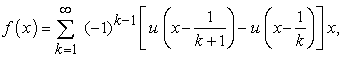which is neither differentiable nor Lipschitzian over I = [0,1]. Indeed, this function, being continuous up to a discrete set of points, i.e. is continuous a.e., should obviously be Riemann integrable. Our purpose here, however, is to arrive at the same conclusion via theorem 1. Hence it can be graphically demonstrated that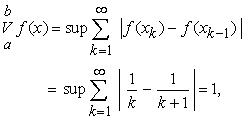(19)

i.e. it is of BV over [0,1]. Obviously,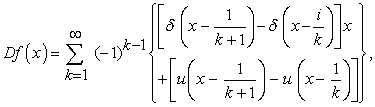and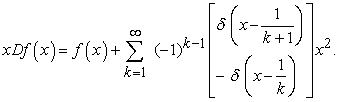Then by (18) we may write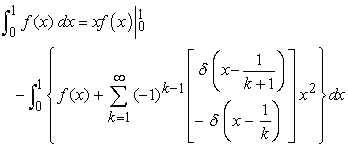Now since x f(x)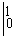= 0, the previous relation becomes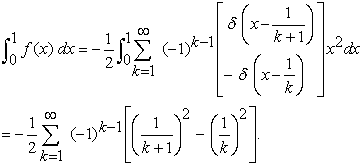Substitution of the identity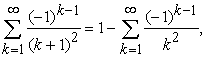in the previous relation reduces it to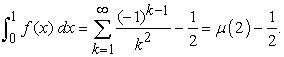The above Dirichlet's Eta function η(s), of argument 2, is related to the associated Riemann Zeta function ξ(2), via η(2) = (1 -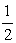) ξ(2). So as ξ(2) = 2/6, 14, we may rewrite the last relation as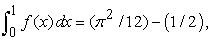i.e. f(x) is Riemann integrable.

3.1. Novel Rational Expansion

One of the rather unexpected applications of theorem1 when integrating certain strongly oscillatory functions in W,1(I;x) is the possibility of generating expansions of some fractions in certain unusual partial fractions. Such a possibility is highlighted in the example that follows.

Example 2. According to Theorem 1, localization of nondifferentiability is not a required restriction. For an illustration, consider a function f(x) defined viz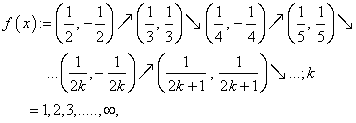(20)

with linear chords, of distinct slopes, between consecutive vertices. This f(x) is a saw-like oscillation which, though continuous, is nondifferentiable at an infinite number of points on I = [0,1]. It can be graphically verified that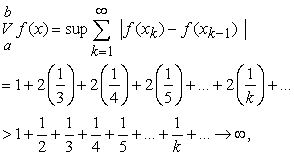i.e. f(x) is not of BV on I. Moreover, the behavior of its chord's slopes as k→∞ is a reason for its non-Lipschitzianity over this I. Nevertheless, it is not difficult to verify the Riemann integrability of this function over I, either directly graphically, or via the application of Theorem 1.

Graphical integration. When integrating the posing saw-like oscillation f(x) on [0,1], we encounter different directions of flow in the oscillation numbers, k, and in values of the x-axis. It turns out to be more convenient to use the map z = 1-x and integrate f(z) over z [0,1]. In fact x = 1/k becomes z =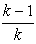. Then for odd k only, i.e. for k = 2m-1; m = 1,2,3,......, z =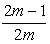. Accordingly, any m-th oscillation on the z-axis becomes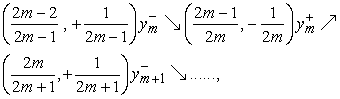(21)

where the middle vertex is below the z-axis,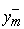=(z) and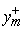=(z) are straight lines with opposite slopes. Direct evaluation of the sum, Am, of the areas of the hanging on the z-axis three triangles, which is the integral of the m-th oscillation, can, by some lengthy calculations, be shown to be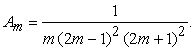(22)

Hence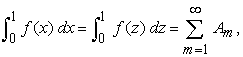(23)

with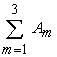= 0.113605,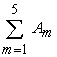= 0.113689, and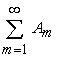≈ 0.115.

Integration via Theorem 1. Hereandneed to be represented analytically viz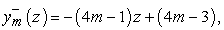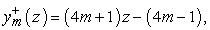to allow for expressing the associated function f(z) as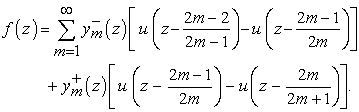(24)

Since z f(z)= 0 , then application of theorem 1 results withwhich can be shown, after some lengthy details, to lead to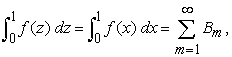(25)

with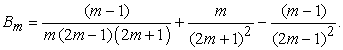(26)

It is straight forward to verify that Bm ≡ Am and that (25) and (27) are identical. Interestingly, however, Bm is not a rational expansion of the rational Am in simple partial fractions viz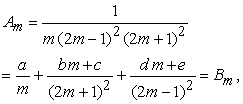(27)

in which a = 1, b = -2, c = -3/2 , d = -2, and e = 3/2, as a must.

Clearly the first term in (26) is not a simple partial fraction for our Am. Hence comparison of (26) and (27) illustrates that Bm, in (26), is remarkably a novel rational expansion of Am, of (23), in unusual partial fractions. This fact can perhaps motivate other "recreational" or unforeseen applications for Theorem 1, along the arguments used in 15, for example.

### 4. Conclusion

The result of this note is meant to complement (and not to replace) the theory of Riemann integration of a.e. continuous functions. It implements for the first time, however, Sobolev space arguments to assert the well known result that BV is not necessary (though sufficient) for Riemann integrability. The distinguishing feature of the function of example 2, from that of example 1, is its non BV on the respective I. It is demonstrated that BV is not necessary for the Riemann integrability of example 2 over [0.1], indicating the surplus in its sufficiency (non-necessity) implied by Theorem 1. The computational potential of Theorem 1, as a technique of integration, raises, according to example 2, an issue that calls for further exploration.

### References

  Royden, H. R, Real analysis, Macmillan, London, 1988. In article  Graves, L. M, “Riemann Integration and Taylor's theorem in general analysis,” Transactions of the AMS, 27. 163-177. 1927. In article View Article  Gordon, R, “Riemann integrability in Banach spaces,” Rocky Mountain Journal of Mathematics, 21(3). 923-949. 1991. In article View Article  Rejouani, R, “On the question of the Riemann integrability of functions with values in a Banach space,” Vestnik Moskov. Univ., Ser. I, Mat. Mech. 26(4). 75-79.1971. In article  McShane, E, A Riemann-type integral that includes Lebesgue-Stieltjes, Bochner and stochastic integrals, Vol.88, AMS, Providence, RI, 1969. In article View Article  Diestel, J. and Uhl, J. J, Vector measures, AMS, Providence, RI, 1977. In article View Article  Kufner, A., John, O. and Fučík, S, Function spaces, Noordhoff, Leyden, 1977. In article  Rudin,W, Principles of mathematical analysis, McGraw-Hill, Auckland, 1964. In article  Titchmarsh,E. C, The theory of functions, Oxford University Press, London,1939. In article  Haidar, N. H. S, “A geometric note on integration,” Computers & Mathematics With Applications, 59(9). 3130-3136. 2010. In article View Article  Hille, E and Philips, R. S, Functional analysis on semigroups, AMS, Providence, RI, 1974. In article  Young, L. C, “An equality of the Hölder type, connected with Stieltjes integration,” Acta Mathematica, 67(1). 251-282. 1936. In article View Article  Kolmogorov, A. N and Fomin, S. V, Introductory real analysis, Dover Publications, 1975. In article  Janke, E., Emde, F. and Lösch, F, Taflen hoherer funktionen, Tubner, Stuttgart, 1960. In article  Haidar, N. H. S, “A rational triplic form for the exponential,” Journal of Computational Analysis and Applications, 4(4). 389-404. 2002. In article View ArticleThis work is licensed under a Creative Commons Attribution 4.0 International License. To view a copy of this license, visit http://creativecommons.org/licenses/by/4.0/